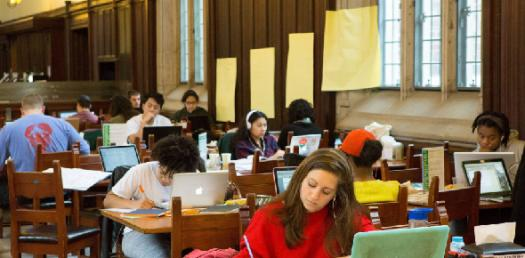# Bio1334 Genetics - Molecular Genetics - Patterns And Principles Of Heredity (Lecture Ten)

6 Questions | Total Attempts: 133SettingsDiagram meiosis, including the behaviour of alleles at a locus during meiosis Demonstrate an understanding of how traits are transmitted from one generation to the next Do monohybrid crosses using the Law of Segregation and basic probabilities Do dihybrid crosses using the Law of Independent Assortment and basic probabilities (using both Punnett Square and ‘branching diagram’ techniques) Use the appropriate vocabulary utilised in genetic crosses

Related Topics
• 1.
Complete the following sentence:Each adult has two genes for each character; different forms of the genes are called _______.
• 2.
True or False?"Meiosis results in the formation of two types of gametes in equal numbers "
• A.

True

• B.

False

• 3.
If two heterozygote parents reproduce, what is the probability their offspring will be homozygous recessive?
• A.

25%

• B.

50%

• C.

75%

• D.

100%